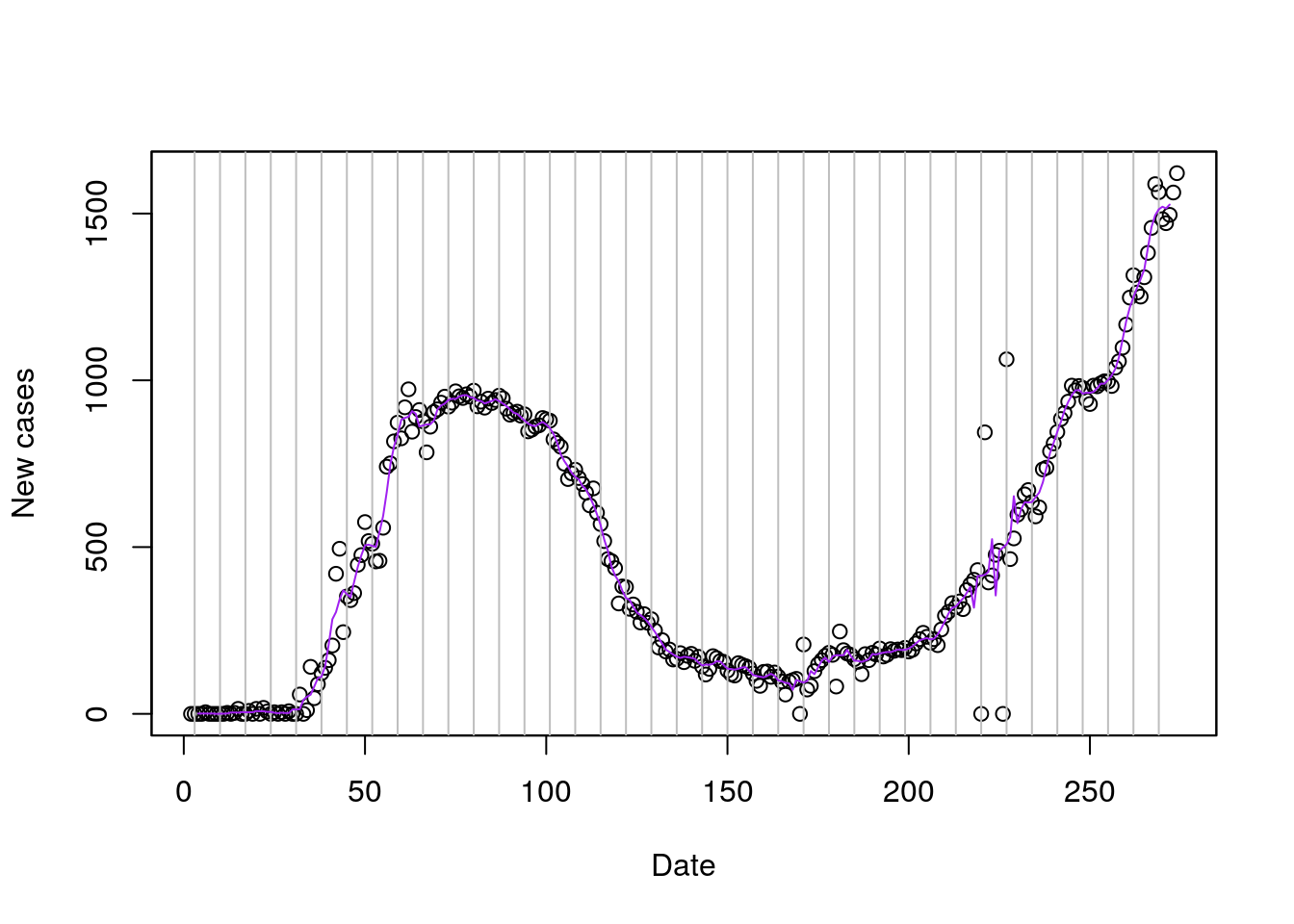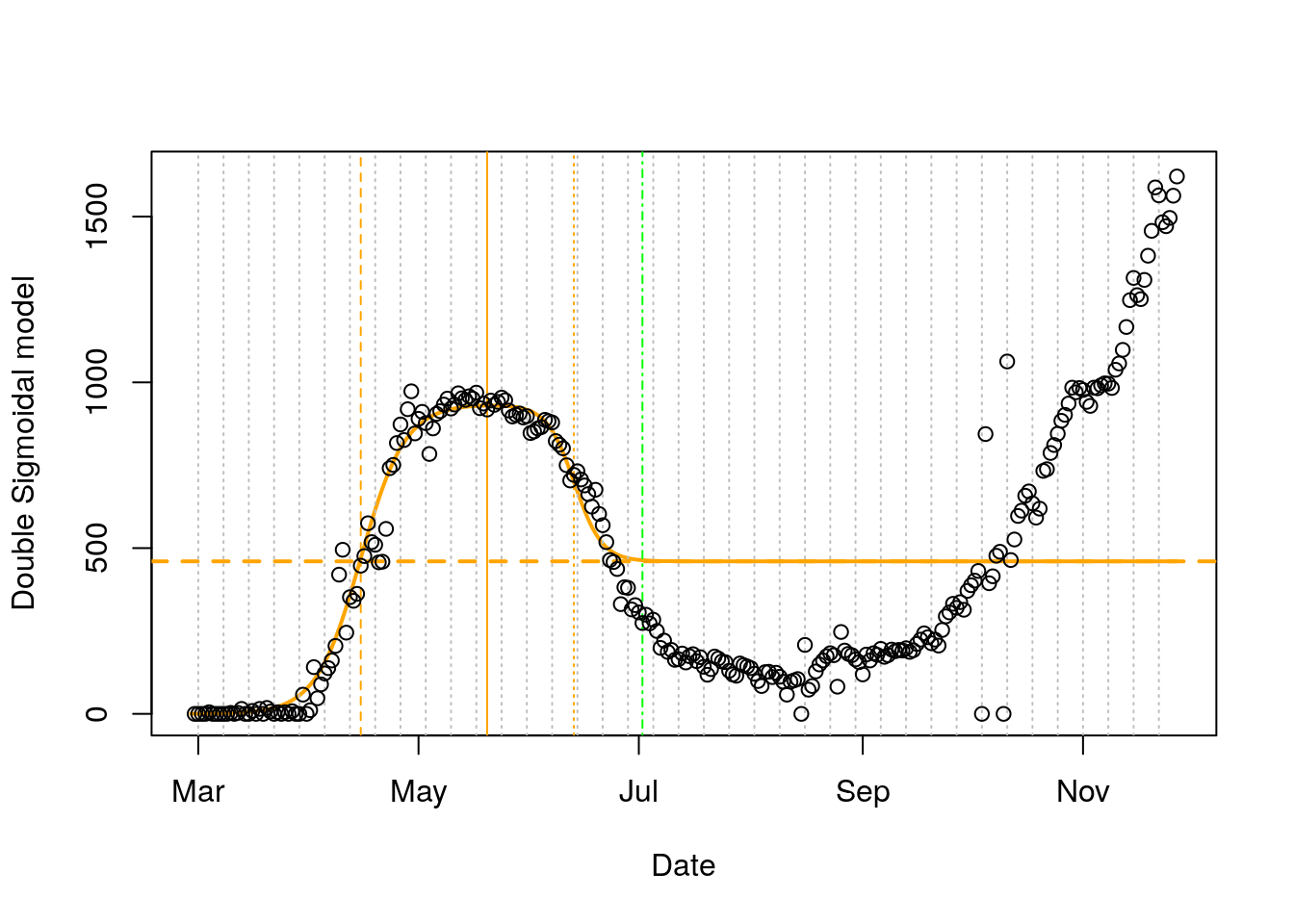Executive Summary: The first wave has ended in Belarus on 2020-07-02 (Day 125). We expect a total of 111,249 cases in the first wave of the COVID-19 pandemic in Belarus, this is an additional 0 cases compared to current case total. New cases have peeked on 2020-05-20 with 930 new cases. Our models assume that after end of the current wave around 460 new cases will appear on an average day, since SARS-CoV-2 is now endemic. Quick, effective, and differentiated mitigation measures need to be in place to prevent a new outbreak.

# 1 New Cases

According to the Johns Hopkins University global COVID-19 data set  a total of 131,633 COVID-19 cases were reported in Belarus on 2020-11-27. This number of cases increased by 1.2 percent over the cumulative total cases known one day earlier. At this rate of cases double every 88 days and the basic reproduction rate $$R_0$$ in Belarus can be estimated at 1, an estimate of the average number of other people infected by a single infected person. Figure 1.1 shows the time line of new cases reported in the country (black points) together with a five day moving average (purple line) that smoothes daily fluctuations in the reporting that are due to fewer capacities on weekends, holidays and other reporting mishaps.Figure 1.1: New cases and five day moving average.

## 1.1 Model for new cases (DSM)

New cases peek after a certain time of growth that follows a sigmoidal function and then recedes to a lower level as the epidemic wave fades out and enters an endemic state. This behavior can be modelled with a double-sigmoid function $$f_{base}$$, that is obtained by the product of two sigmoidal functions .

$f_{base}(t) = \frac{1}{ 1+e^{-\alpha_{i} * (t - t_{i})} } * \frac{1}{ 1+e^{-\alpha_{d} * (t - t_{d})} }$

This function has a maximum $$f_{max} = max(f_{base}(t_{max}))$$ at $$t_{max}$$.

The model can obtain predictions related to an observed maximum of new cases $$I_{max_{d}}$$ and an endemic number of daily cases $$I_{final_{d}}$$ as an asymptote (that is ideally 0). Let $$f_{d}(t)$$ be a piecewise function that switches between the growth and decay phase at $$t_{max}$$, such that

\begin{aligned}f_{d}(t) = \left\{ \begin{array}{cc} \frac{I_{max_{d}}}{f_{max}} * f_{base}(t) & \hspace{5mm} t \leq t_{max} \\ \frac{I_{max_{d}} - I_{final_{d} }}{f_{max}} * f_{base}(t) + I_{final_{d}} & \hspace{5mm} t > t_{max} \\ \end{array} \right. \end{aligned}

where $$t_{i}$$ is the midpoint and $$\alpha_{i}$$ the slope of the increase of cases and $$t_{d}$$ is the midpoint and $$\alpha_{d}$$ is the slope of the decrease in cases. $$I_{max_{d}}$$ the peak of new cases and $$I_{final_{d}}$$ the asymptotic final value of new cases at the end of the infections wave. Figure 1.2 shows the current double-sigmoidal model ( orange line) that is fitted for the new case time series of Belarus, where the vertical dashed line highlights the midpoint $$t_i$$ of the growth phase, the vertical solid line indicates the peak of new infections $$t_max$$ and the vertical dotted line the midpoint $$t_d$$ of the decay phase. The dashed horizontal line marks the estimated asymptote of endemic new cases $$I_{final_{d}}$$ as this infection wave ends. We pragmatically assume that the first wave ends, when 99 percent of the decrease has happened. The dashed green line marks this date.Figure 1.2: Double sigmoidal model (DSM) for new cases.

## 1.2 Reliability of the DSM

Since the model requires six parameters, it also requires more data points. Hence, it is only feasible to fit this model in later phases of the pandemic (ideally after the peak of daily infections occured) and the model is highly sensitive to new data, particularly with respect to finding the final asymptote$$I_{final_{d}}$$as the following comparison of the model shows. Nevertheless the model renders interesting characteristica of the past developments.

Model for Daily Increases Current Estimate Last Week Estimate Delta (absolute)
$$I_{max_{d}}$$ Peak new cases 930 929 1
$$I_{final_{d}}$$ Endemic new cases 460 417 43
$$\alpha_{i}$$ Slope of growth phase 0.16 0.16 0
$$t_{i}$$ Midpoint of growth phase 2020-04-15 (Day 48) 2020-04-15 (Day 48) 0
$$t_{max}$$ Peak day 2020-05-20 (Day 83) 2020-05-20 (Day 83) 0
$$\alpha_{d}$$ Slope of decay phase 0.26 0.25 0.01
$$t_{d}$$ Midpoint of decay phase 2020-06-13 (Day 107) 2020-06-14 (Day 108) -1
First wave ends 2020-07-02 (Day 125) 2020-07-02 (Day 125) 0

# 2 Cumulative cases

The cumulative total of COVID-19 cases in Belarus is the number of all COVID-19 cases known up to yesterday and includes all patients already recovered from the infection. This corresponds to the Infected (I) number in the standard epidemiological SIR model. Figure 2.1 shows the data points of the time series together with a Gompertz model for the cumulative cases (red line) as well as the cumulated double-sigmoidal model (orange line). The dashed horizontal lines show the expected maximum number of cases of the respective models. Note: The cumulated double-sigmoidal model does not have an asymptote, since $$I_{final_{d}}$$ is not zero, the line can be considered as the number of infections when the infective wave ends and the endemic state starts.